# Chapter 3 Differential Motions and Velocities 3 1

• Slides: 17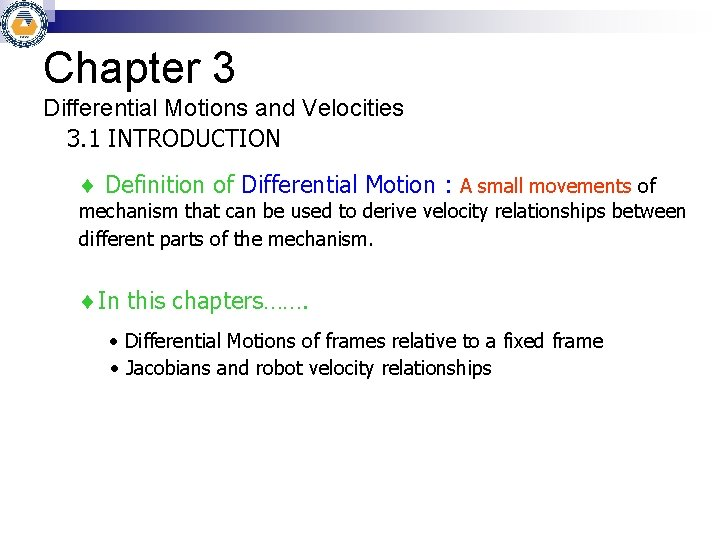Chapter 3 Differential Motions and Velocities 3. 1 INTRODUCTION Definition of Differential Motion : A small movements of mechanism that can be used to derive velocity relationships between different parts of the mechanism. In this chapters……. Differential Motions of frames relative to a fixed frame Jacobians and robot velocity relationships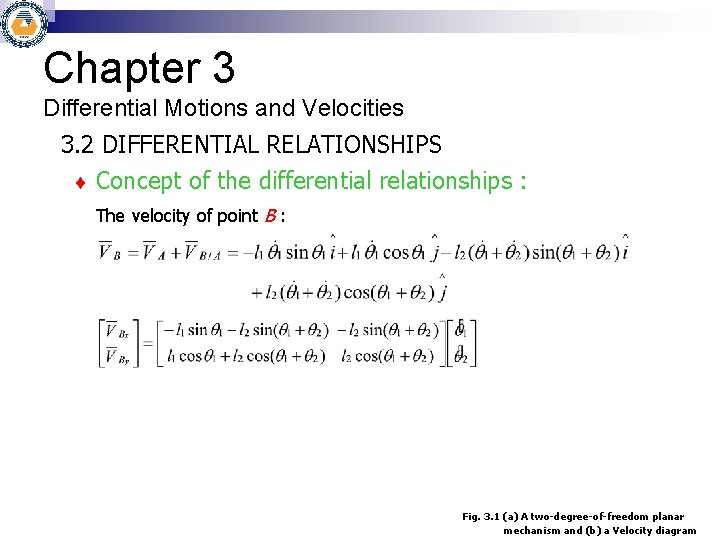Chapter 3 Differential Motions and Velocities 3. 2 DIFFERENTIAL RELATIONSHIPS Concept of the differential relationships : The velocity of point B : Fig. 3. 1 (a) A two-degree-of-freedom planar mechanism and (b) a Velocity diagram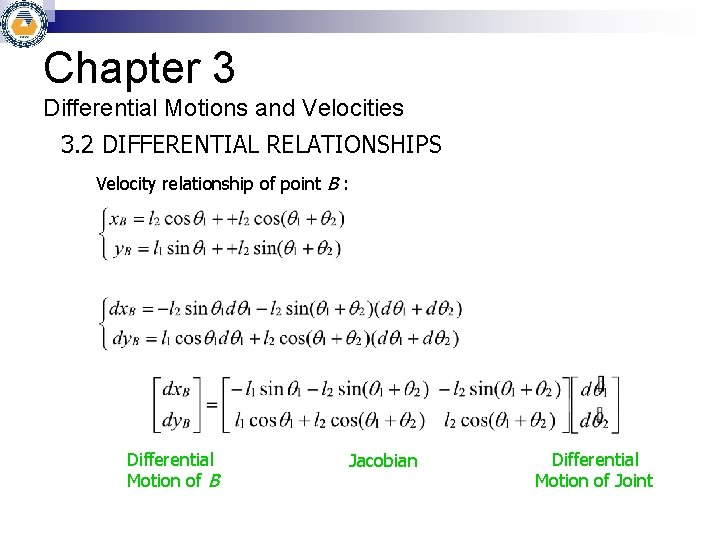Chapter 3 Differential Motions and Velocities 3. 2 DIFFERENTIAL RELATIONSHIPS Velocity relationship of point B : Differential Motion of B Jacobian Differential Motion of Joint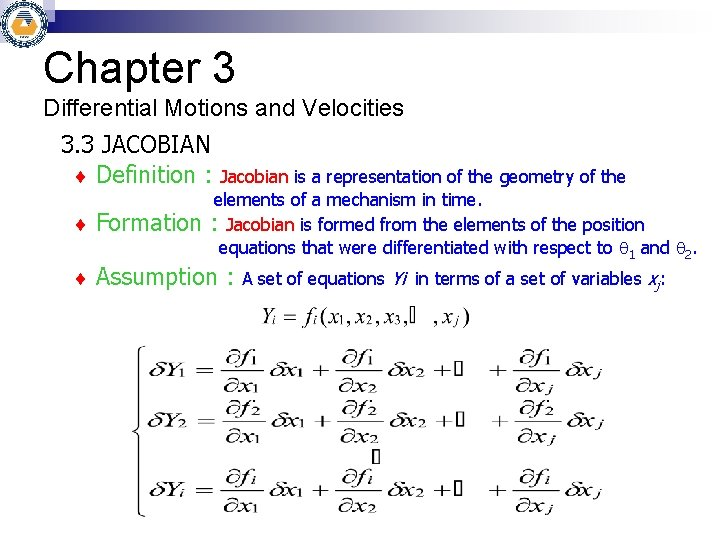Chapter 3 Differential Motions and Velocities 3. 3 JACOBIAN Definition : Jacobian is a representation of the geometry of the elements of a mechanism in time. : Jacobian is formed from the elements of the position equations that were differentiated with respect to 1 and 2. Formation Assumption : A set of equations Yi in terms of a set of variables xj:Chapter 3 Differential Motions and Velocities 3. 3 JACOBIAN Matrix Representation Differentiating the position equations of a robot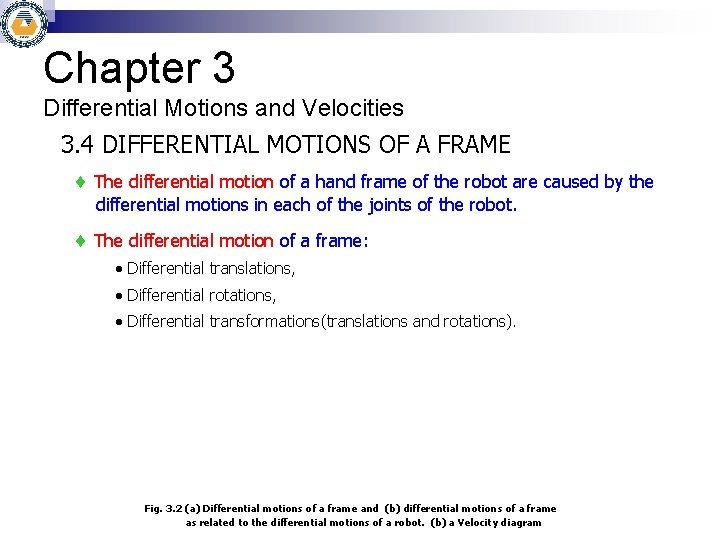Chapter 3 Differential Motions and Velocities 3. 4 DIFFERENTIAL MOTIONS OF A FRAME The differential motion of a hand frame of the robot are caused by the differential motions in each of the joints of the robot. The differential motion of a frame: Differential translations, Differential rotations, Differential transformations(translations and rotations). Fig. 3. 2 (a) Differential motions of a frame and (b) differential motions of a frame as related to the differential motions of a robot. (b) a Velocity diagram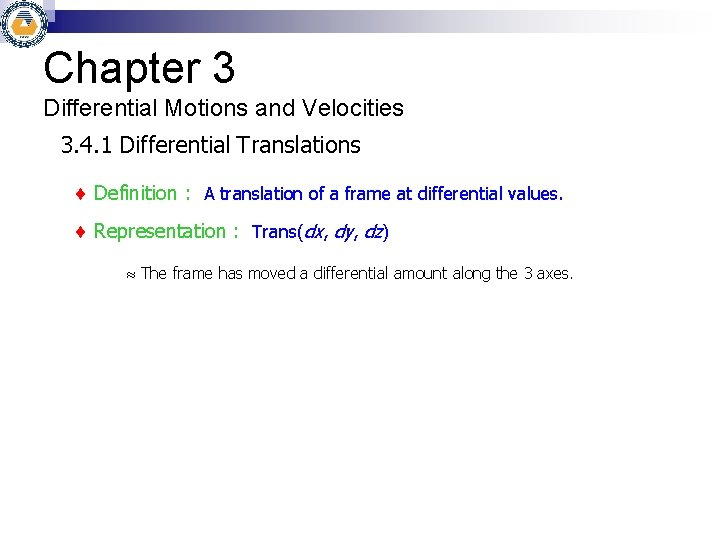Chapter 3 Differential Motions and Velocities 3. 4. 1 Differential Translations Definition : A translation of a frame at differential values. Representation : Trans(dx, dy, dz) The frame has moved a differential amount along the 3 axes.Chapter 3 Differential Motions and Velocities 3. 4. 2 Differential rotations Definition : A small rotation of a frame at differential values. Representation : Rot(k, d ) The frame has rotated an angle of d about an axis Differential rotation about x, y, z-axis is x, y, z, respectively.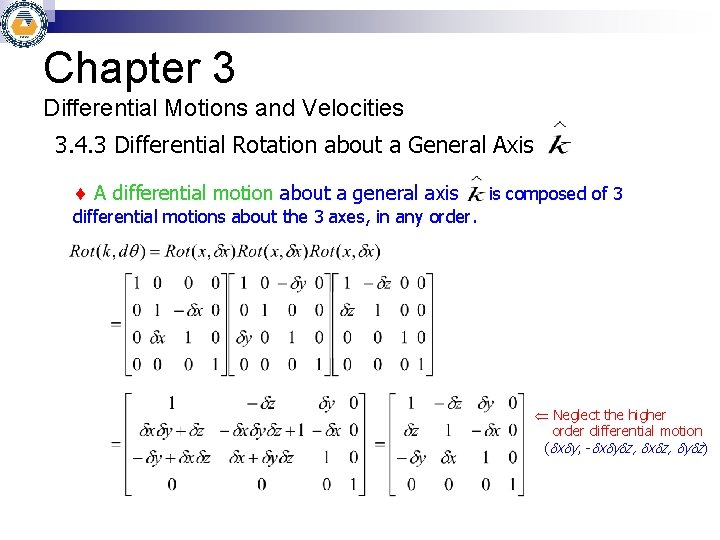Chapter 3 Differential Motions and Velocities 3. 4. 3 Differential Rotation about a General Axis A differential motion about a general axis is composed of 3 differential motions about the 3 axes, in any order. Ü Neglect the higher order differential motion ( x y, - x y z, x z, y z)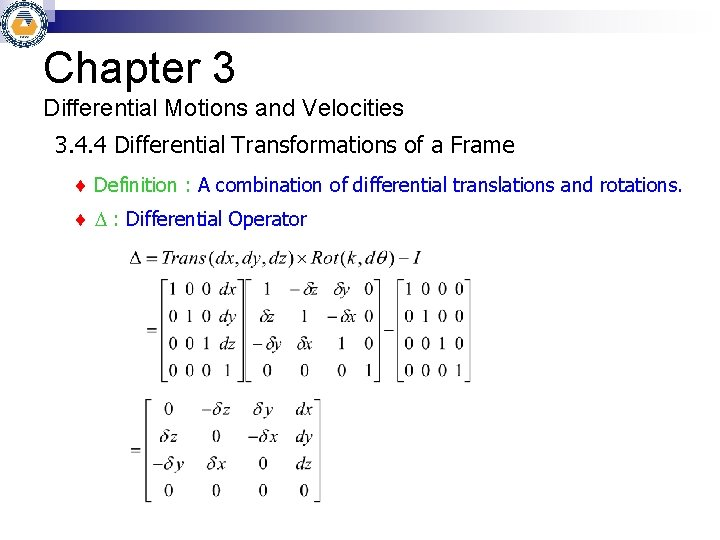Chapter 3 Differential Motions and Velocities 3. 4. 4 Differential Transformations of a Frame Definition : A combination of differential translations and rotations. : Differential Operator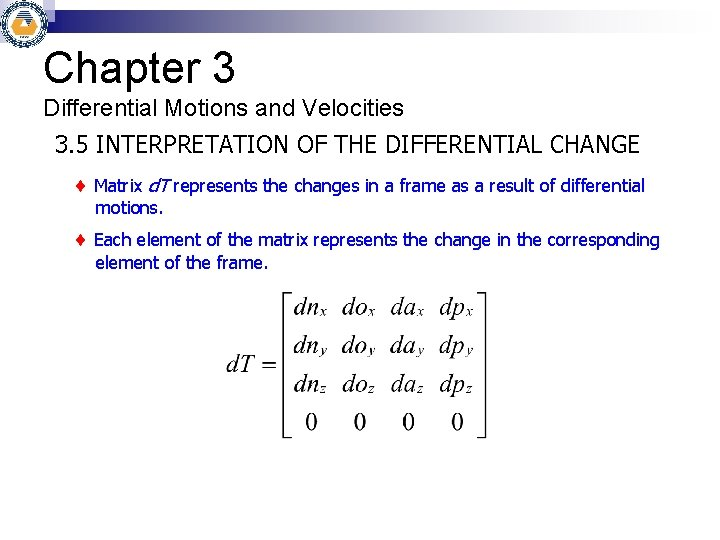Chapter 3 Differential Motions and Velocities 3. 5 INTERPRETATION OF THE DIFFERENTIAL CHANGE Matrix d. T represents the changes in a frame as a result of differential motions. Each element of the matrix represents the change in the corresponding element of the frame.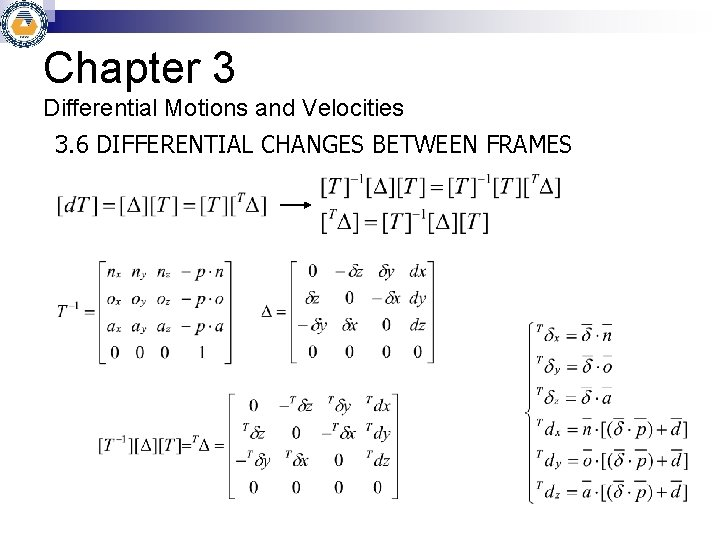Chapter 3 Differential Motions and Velocities 3. 6 DIFFERENTIAL CHANGES BETWEEN FRAMES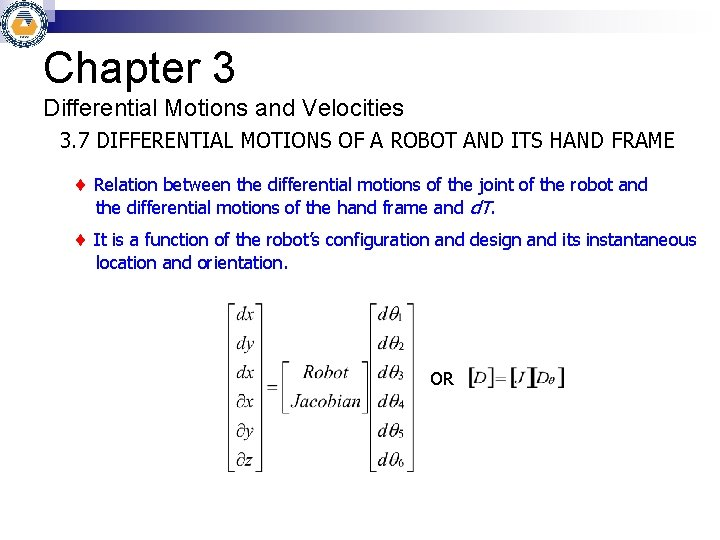Chapter 3 Differential Motions and Velocities 3. 7 DIFFERENTIAL MOTIONS OF A ROBOT AND ITS HAND FRAME Relation between the differential motions of the joint of the robot and the differential motions of the hand frame and d. T. It is a function of the robot’s configuration and design and its instantaneous location and orientation. OR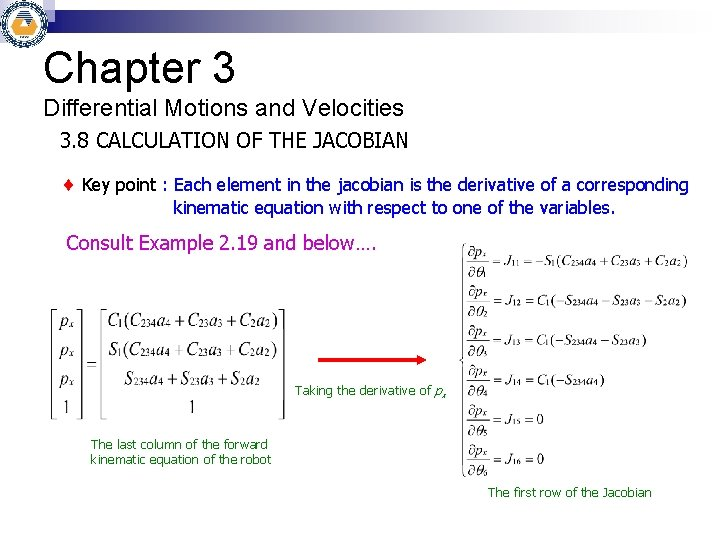Chapter 3 Differential Motions and Velocities 3. 8 CALCULATION OF THE JACOBIAN Key point : Each element in the jacobian is the derivative of a corresponding kinematic equation with respect to one of the variables. Consult Example 2. 19 and below…. Taking the derivative of px The last column of the forward kinematic equation of the robot The first row of the Jacobian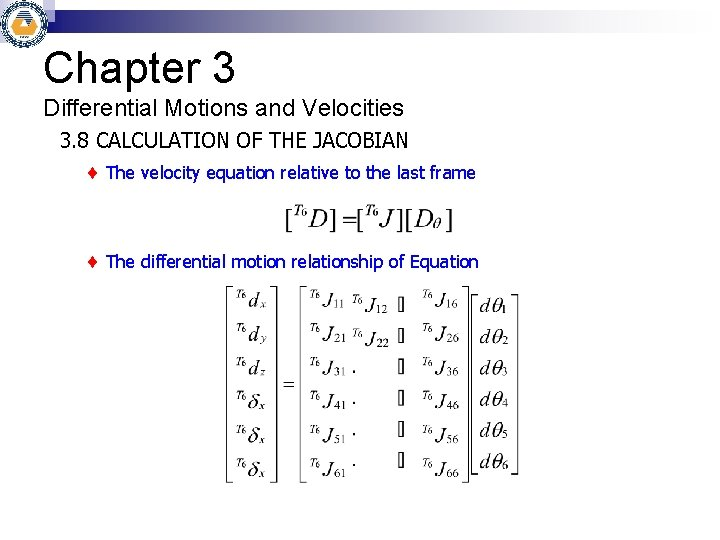Chapter 3 Differential Motions and Velocities 3. 8 CALCULATION OF THE JACOBIAN The velocity equation relative to the last frame The differential motion relationship of EquationChapter 3 Differential Motions and Velocities 3. 9 HOW TO RELATE THE JACOBIAN AND THE DIFFERENTIAL OPERATOR The differential motions of the robot’s joints are ultimately related to the hand frame of the robot. Equ. 3. 10 and Jacobian calculate [D] matrix [D] contains differential motions of the hand, dx, dy, dz, x, y, z. Equ. 3. 15 used to calculate d. T Equ. 3. 24 and Jacobian calculate [T 6 D] matrix [D] contains differential motions of the hand, T 6 dx, T 6 dy, T 6 dz, T 6 x, T 6 y, T 6 z. Equ. 3. 19 used to calculate d. TChapter 3 Differential Motions and Velocities 3. 10 INVERSE JACOBIAN Inverse Jacobian used to calculate the differential motions needed at the joints of the robot for a desired hand differential motion. Inverse Jacobian calculates how fast each joint must move so that the robot’s hand will yield a desired differential motion or velocity. To make sure the robot follows a desired path, the joint velocities must be calculated continuously in order to ensure that the robot’s hand maintains a desired velocity.